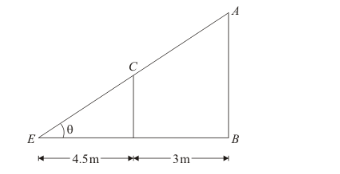# If a 1.5 m tall girl stands at a distance of 3 m from a lamp-post

Question:

If a 1.5 m tall girl stands at a distance of 3 m from a lamp-post and casts a shadow of length 4.5 m on the ground, then the height of the lamp-post is

(a) 1.5 m

(b) 2 m

(c) 2.5 m

(d) 2.8 m

Solution:

Let AB be the lamp post and CD =1.5 m be the girl.

The given information can be represented asHere, shadow of girl is DE = 4.5 m and BD = 3 m.

In $\triangle C D E, \tan \theta=\frac{C D}{D E}=\frac{1.5}{4.5}$....(1)

In $\triangle A B E, \tan \theta=\frac{A B}{B E}=\frac{A B}{4.5+3}=\frac{A B}{7.5}$.....(2)

From $(1)$ and $(2)$,

$\frac{1.5}{4.5}=\frac{A B}{7.5}$

$\Rightarrow A B=\frac{1.5 \times 7.5}{4.5}=2.5 \mathrm{~m}$

Therefore, height of the lamp post is 2.5 m

Hence the correct option is.